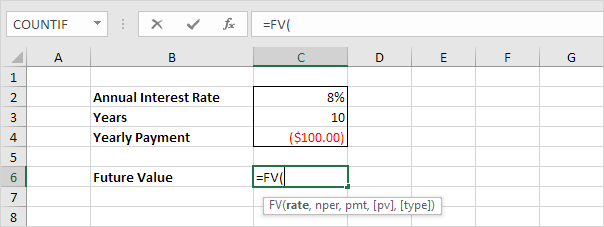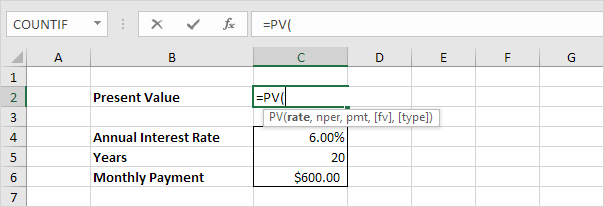# Investment or Annuity

This example teaches you how to calculate the future value of an investment or the present value of an annuity.

Tip: when working with financial functions in Excel, always ask yourself the question, am I making a payment (negative) or am I receiving money (positive)?

### Investment

Assume that at the end of every year, you deposit \$100 into a savings account. At an annual interest rate of 8%, how much will your investment be worth after 10 years?

1. Insert the FV (Future Value) function.2. Enter the arguments.In 10 years time, you pay 10 * \$100 (negative) = \$1000, and you'll receive \$1,448.66 (positive) after 10 years. The higher the interest, the faster your money grows.

Note: the last two arguments are optional. If omitted, Pv = 0 (no present value). If Type is omitted, it is assumed that payments are due at the end of the period.

### Annuity

Assume you want to purchase an annuity that will pay \$600 a month, for the next 20 years. At an annual interest rate of 6%, how much does the annuity cost?

1. Insert the PV (Present Value) function.2. Enter the arguments.You need a one-time payment of \$83,748.46 (negative) to pay this annuity. You'll receive 240 * \$600 (positive) = \$144,000 in the future. This is another example that money grows over time.

Note: we receive monthly payments, so we use 6%/12 = 0.5% for Rate and 20*12 = 240 for Nper. The last two arguments are optional. If omitted, Fv = 0 (no future value). If Type is omitted, it is assumed that payments are due at the end of the period. This annuity does not take into account life expectancy, inflation etc.

Go to Next Chapter: Statistical Functions